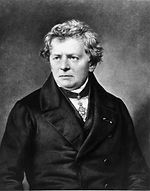top of page# What is 1 Ohm ?

The dimension of an electric resistance is the “Ohm”.
It is abbreviated with the Greek letter Omega ΩGeorg Simon Ohm

16 March 1789 – 6 July 1854

•     German physicist and mathematician

•     Ohm’s Law  R = U  / I  published 1827 in
“The Galvanic Circuit Investigated

Mathematically”

•     The unit of resistance was named “Ohm”

in his honourMr Ohm wanted to develop a mathematical relation in order to calculate the “intensity of flowing electricity” (= electric current) as a function of the material and the dimensions of the wire. What he found is one of the very basic laws of electrical engineering. It seems almost trivial to us but it wasn't at all at the time:
The higher the voltage U and the smaller the resistance R, the larger is the electric current I

I = U/R   or   U = R·I    or    R = U/I

The dimension:  [ R ] = Ohm = Ω = V/A

Ohm stipulated that the relation U/I  i.e. the resistance was an independent constant. Insofar he was not quite right as we know today that most resistances are not constant but most often depend on the temperature or many other things.

The diagram shows how a currentthat is flowing from A to B

develops a voltage U  across the resistance R .

For our particular application in piezo-electrics we talk about extremely high resistances. As we have seen before a piezo element is a good insulator with an internal resistance from pole to pole (from electrode to electrode) in the order of 10¹²  Ohms or more.

In a “normal electronics” environment we would simply neglect such high resistances but in a “piezo electric environment” we need to care about.

Normally we count in logarithmic terms like 10⁷, 10⁸, 10⁹  etc. We remember that 10⁹   would mean a one with 9 zeros.

Sometimes the decimal multipliers  Mega- ; Giga- ; Tera- are used for 10⁶, 10⁹, 10¹²  respectively.

bottom of page